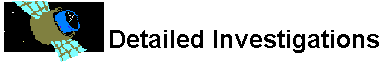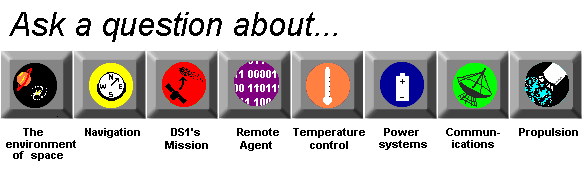# Power System

## What are volts and amperes?

Volts and amperes are measures of electricity.

A volt is the unit of electric potential difference, or the size of the force that sends the electrons through a circuit.

An ampere is the unit used to measure electric current. Current is a count of the number of electrons flowing through a circuit. One amp is the amount of current produced by a force of one volt acting through the resistance of one ohm.

(An ohm is a way of measuring resistance. A certian length of copper wire, which is a good conductor, has a resistance of .0000017 ohms, while the same length of Sulfur, which is a very poor conductor, is much more resistant. It has a resistance of 200,000,000,000,000,000 ohms!)How does power get distributed in DS1?Where does DS1 get its electricity?What's a circuit?What's an electron?What's electron flow?What is resistance?How much power does DS1 use? How much power does it produce?What are the new low power electronics on DS1?What's a resistor?